### Tangents And Secants To A Circle Class 10th Mathematics AP Board Solution

##### Question 1.A chord of a circle of radius 10 cm. subtends a right angle at the centre. Find the area of the corresponding :(use π = 3.14)i. Minor segmentii. Major segmentAnswer: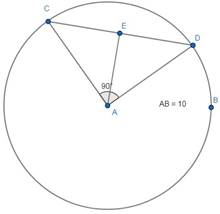i. Let Major segment A1 minor segment be A2∠A = 90°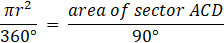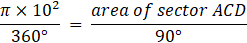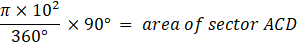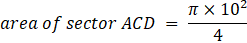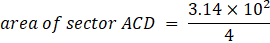area of sector ACD = 78.5cm2PythagorasAC2 = CD2 + AD2AC2 = 102 + 102AC2 = 200AC = 10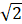Height of triangle =Cos 45° =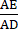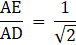AE =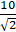Area of minor segment = Area of sector ACD – Area ΔACD= 78.5 –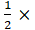10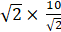= 78.5-50= 28.5 cm2ii. Major segment = Area of circle – minor segment= π × r2 –minor segment= π × 102 –28.5= 314-28.5= 285.5cm2Question 2.A chord of a circle of radius 12 cm. subtends an angle of 120o at the centre. Find the area of the corresponding minor segment of the circle(use π = 3.14 and √3 = 1.732)Answer: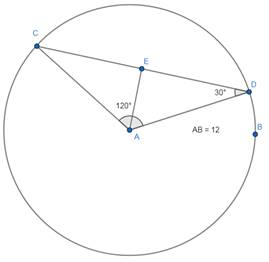Let Major segment A1 minor segment be A2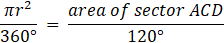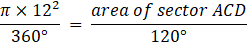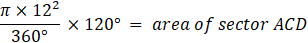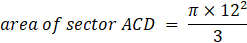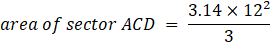area of sector ACD = 150.72 sq.cmSin 30° =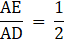AE = 6cmCos 30° =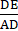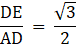DE = 1.732 × 6DE = 10.392cmCD = 2 × 10.392 = 20.784cmArea of minor segment = Area of sector ACD – Area ΔACD= 150.72 –20.784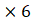= 1550.72-62.352= 88.44 cm2Question 3.A car has two wipers which do not overlap. Each wiper has a blade of length 25 cm. sweeping through an angle of 115o. Find the total area cleaned at each sweep of the blades.(use π = 22/7)Answer:Radius = 25cmAngle = 115°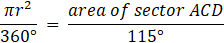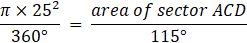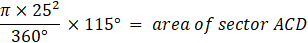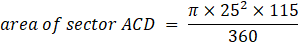Area of sector ACD = 627.22cm2For 2 such wipers: 2 × 627.22Area = 1254.45 cm2Question 4.Find the area of the shaded region in figure, where ABCD is a square of side 10 cm. and semicircles are drawn with each side of the square as diameter(use π = 3.14)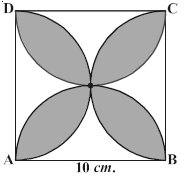Answer:Consider midpoint side AB, it forms Smaller square of size 5cmFor this smaller squareArea of shaded region= Area of 1st quadrant + area of 2nd quadrant –area of squareArea of both quadrants is same∴ Area of shaded region= 2 × Area of quadrant - area of square= 2 ×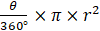– r × r= 2 ×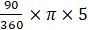– 5 × 5= 2 ×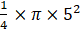– 5 × 5= 14.25 cm2Area of total shaded region = 4 × 14.25= 57 cm2Question 5.Find the area of the shaded region in figure, if ABCD is a square of side 7 cm. and APD and BPC are semicircles.(use π = 22/7)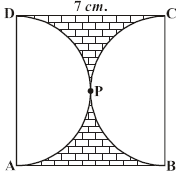Answer:Area of shaded region = Area of square – area of 2 semicirclesArea of square = 7 × 7 = 49 sq.cmArea of semicircles =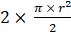= π × r2= π × 3.52= 38.5 sq.cmArea of shaded region = 49-38.5 = 10.5 cm2Question 6.In figure, OACB is a quadrant of a circle with centre O and radius 3.5 cm. If OD = 2 cm., find the area of the shaded region.(use π = 22/7)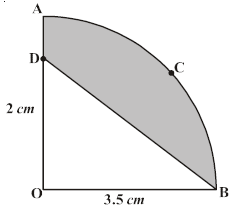Answer:Area of Shaded region = Area of sector OABC-Area of ΔDOBOB = OA = 3.5cm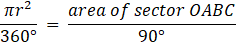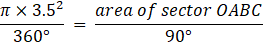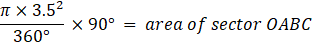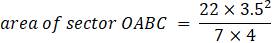area of sector OABC = 9.625 cm2Area of ΔDOB =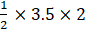= 3.5 cm2Area of Shaded region = 9.625-3.5= 6.125 cm2Question 7.AB and CD are respectively arcs of two concentric circles of radii 21 cm. and 7 cm. with centre O (See figure). If ∠AOB = 30°, find the area of the shaded region.(use π = 22/7)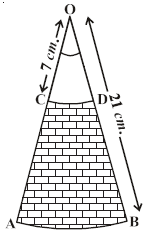Answer:Area of shaded region = Area(Sector OAB)-Area(Sector OCD)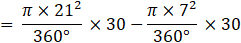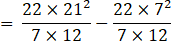= 102.67 cm2Question 8.Calculate the area of the designed region in figure, common between the two quadrants of the circles of radius 10 cm. each.(use π = 3.14)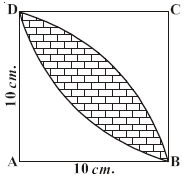Answer:Area of shaded region= Area of 1st quadrant + area of 2nd quadrant –area of squareArea of both quadrants is same∴ Area of shaded region= 2 × Area of quadrant - area of square= 2 ×– r × r= 2 ×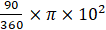– 10 × 10= 2 ×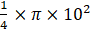– 10 × 10= 57.07 cm2

PDF FILE TO YOUR EMAIL IMMEDIATELY PURCHASE NOTES & PAPER SOLUTION. @ Rs. 50/- each (GST extra)

HINDI ENTIRE PAPER SOLUTION

MARATHI PAPER SOLUTION

SSC MATHS I PAPER SOLUTION

SSC MATHS II PAPER SOLUTION

SSC SCIENCE I PAPER SOLUTION

SSC SCIENCE II PAPER SOLUTION

SSC ENGLISH PAPER SOLUTION

SSC & HSC ENGLISH WRITING SKILL

HSC ACCOUNTS NOTES

HSC OCM NOTES

HSC ECONOMICS NOTES

HSC SECRETARIAL PRACTICE NOTES

# 2019 Board Paper Solution

HSC ENGLISH SET A 2019 21st February, 2019

HSC ENGLISH SET B 2019 21st February, 2019

HSC ENGLISH SET C 2019 21st February, 2019

HSC ENGLISH SET D 2019 21st February, 2019

SECRETARIAL PRACTICE (S.P) 2019 25th February, 2019

HSC XII PHYSICS 2019 25th February, 2019

CHEMISTRY XII HSC SOLUTION 27th, February, 2019

OCM PAPER SOLUTION 2019 27th, February, 2019

HSC MATHS PAPER SOLUTION COMMERCE, 2nd March, 2019

HSC MATHS PAPER SOLUTION SCIENCE 2nd, March, 2019

SSC ENGLISH STD 10 5TH MARCH, 2019.

HSC XII ACCOUNTS 2019 6th March, 2019

HSC XII BIOLOGY 2019 6TH March, 2019

HSC XII ECONOMICS 9Th March 2019

SSC Maths I March 2019 Solution 10th Standard11th, March, 2019

SSC MATHS II MARCH 2019 SOLUTION 10TH STD.13th March, 2019

SSC SCIENCE I MARCH 2019 SOLUTION 10TH STD. 15th March, 2019.

SSC SCIENCE II MARCH 2019 SOLUTION 10TH STD. 18th March, 2019.

SSC SOCIAL SCIENCE I MARCH 2019 SOLUTION20th March, 2019

SSC SOCIAL SCIENCE II MARCH 2019 SOLUTION, 22nd March, 2019

XII CBSE - BOARD - MARCH - 2019 ENGLISH - QP + SOLUTIONS, 2nd March, 2019

# HSCMaharashtraBoardPapers2020

(Std 12th English Medium)

HSC ECONOMICS MARCH 2020

HSC OCM MARCH 2020

HSC ACCOUNTS MARCH 2020

HSC S.P. MARCH 2020

HSC ENGLISH MARCH 2020

HSC HINDI MARCH 2020

HSC MARATHI MARCH 2020

HSC MATHS MARCH 2020

# SSCMaharashtraBoardPapers2020

(Std 10th English Medium)

English MARCH 2020

HindI MARCH 2020

Hindi (Composite) MARCH 2020

Marathi MARCH 2020

Mathematics (Paper 1) MARCH 2020

Mathematics (Paper 2) MARCH 2020

Sanskrit MARCH 2020

Sanskrit (Composite) MARCH 2020

Science (Paper 1) MARCH 2020

Science (Paper 2)

Geography Model Set 1 2020-2021

MUST REMEMBER THINGS on the day of Exam

Are you prepared? for English Grammar in Board Exam.

Paper Presentation In Board Exam

How to Score Good Marks in SSC Board Exams

Tips To Score More Than 90% Marks In 12th Board Exam

How to write English exams?

How to prepare for board exam when less time is left

How to memorise what you learn for board exam

No. 1 Simple Hack, you can try out, in preparing for Board Exam

How to Study for CBSE Class 10 Board Exams Subject Wise Tips?

JEE Main 2020 Registration Process – Exam Pattern & Important Dates

NEET UG 2020 Registration Process Exam Pattern & Important Dates

How can One Prepare for two Competitive Exams at the same time?

8 Proven Tips to Handle Anxiety before Exams!